# Solve equations using addition and subtractionPage 1

#### WATCH ALL SLIDES

Slide 1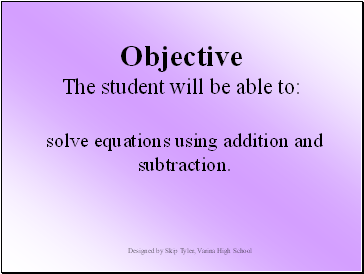## Solve equations using addition and subtraction

Objective The student will be able to:

.

Designed by Skip Tyler, Varina High School

Slide 2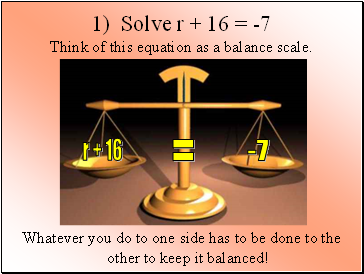1) Solve r + 16 = -7

Think of this equation as a balance scale.

Whatever you do to one side has to be done to the other to keep it balanced!

Slide 3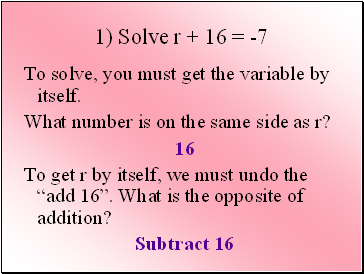1) Solve r + 16 = -7

To solve, you must get the variable by itself.

What number is on the same side as r?

16

To get r by itself, we must undo the “add 16”. What is the opposite of addition?

Subtract 16

Slide 4- 16 -16

r = -23

-23 + 16 = -7

1) Solve r + 16 = -7

Draw “the river” to separate the equation into 2 sides

Subtract 16 from both sides

Simplify vertically

Slide 5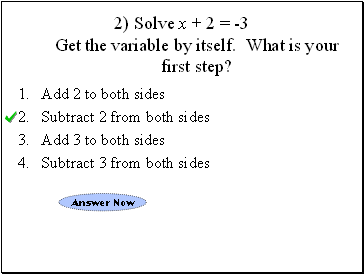2) Solve x + 2 = -3 Get the variable by itself. What is your first step?

Subtract 2 from both sides

Subtract 3 from both sides

Slide 6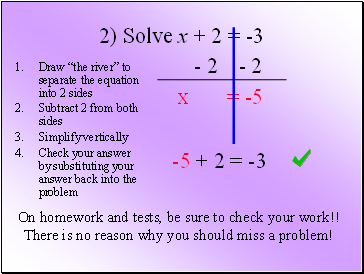2) Solve x + 2 = -3

- 2 - 2

x = -5

-5 + 2 = -3

Draw “the river” to separate the equation into 2 sides

Subtract 2 from both sides

Simplify vertically

On homework and tests, be sure to check your work!!

There is no reason why you should miss a problem!

Slide 73) Solve 8 = m - 3

m = 5

m = 11

m = 24

m = 8/3

Slide 8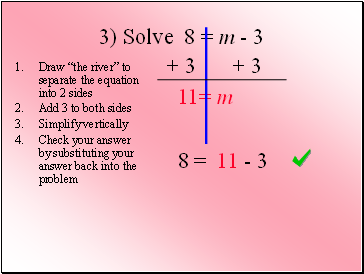3) Solve 8 = m - 3

+ 3 + 3

11= m

8 = 11 - 3

Draw “the river” to separate the equation into 2 sides

Simplify vertically

Slide 9When solving equations, we want to eliminate double signs.

y + (-3) = 8

is rewritten as

y – 3 = 8

p – (-5) = 6

is rewritten as

p + 5 = 6

As a general rule, replace “+ (- )” with “–” and “– (- )” with “+”. This will make things less confusing in the future!

Slide 10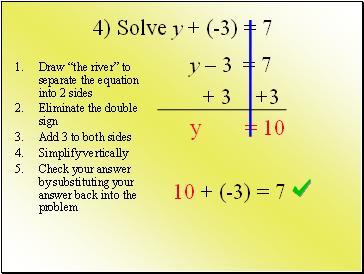4) Solve y + (-3) = 7

y – 3 = 7

+ 3 +3

Go to page:
1  2